### Home > A2C > Chapter Ch11 > Lesson 11.2.5 > Problem11-106

11-106.
1. The graph of f(x) = x2 − 3x − 4 is shown below. Use the graph to solve: Homework Help ✎

 f (x) = 0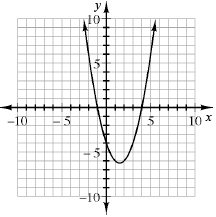f (x) ≥ 0 f (x) ≤ 0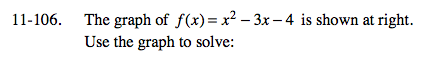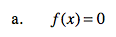Where on the graph is y = 0?

x = −1, x = 4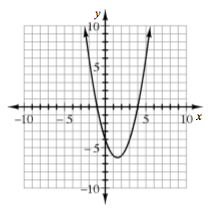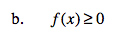Where on the graph are the y-values greater than or equal to 0?

x ≥ 4 or x ≤ −1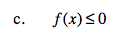See part (b).